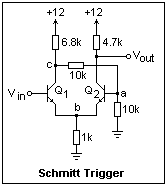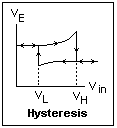## Comparators and Schmitt Triggers

### Comparators and Hysteresis

If the inverting input of an op-amp is higher in voltage than the noninverting input, the output saturates negatively, while if the reverse is the case, it saturates postively. Therefore, the op-amp can detect whether one node is higher in voltage than another, often a useful function. However, an op-amp is not really intended for this kind of duty, and there are some inconveniences, such as the output saturation. In spite of this, op-amps can sometimes be used for the purpose, especially the single-supply op-amps that will go to V-. There are, however, circuits specially built for use as comparators, such as the LM311 and its FET-input sister, the LF311. We'll use the LM311 as the example here. It might be well to say that it is not an op-amp, and cannot be used as an op-amp. Among other things, it would not be stable.An LM311 in a comparator circuit is shown at the right, with the pin numbers for the connections. The open-collector output is at pin 7, and the ground for this transistor is at pin 1. It is shown pulled up to the logic voltage of +5 with a 1k resistor, which is typical. The output of the LM311 can sink 8 mA. A comparator's output is essentially binary, YES or NO. Pin 4 is V- and pin 8 is V+. The total supply voltage can go up to 36 V. If the comparator threshold is above ground by a volt or so, V- can simply be connected to ground, as here. The supply voltage to the LM311 does not have to be the same as the logic supply. Pins 5 and 6 should be shorted together if not used as a balance adjustment (like the nulling of an op-amp). Pin 2 is the noninverting input, while pin 3 is the inverting input. It is usual to establish the reference voltage Vr at pin 2 by a voltage divider, as shown. The reference voltage, or threshold, is about 6 V here.

The 100k resistor from the output to pin 2 provides positive feedback. When the output goes low, the threshold is reduced a little, and when it goes high, the threshold is raised a little. These changes are opposite in direction to the change in the voltage at pin 3 that produced them, so they furnish a "snap" action to the comparator. The fractional change in the threshold is roughly the value of the divider resistors in parallel divided by the feedback resistor, here 5/100 or 0.05. With a 5 V supply, this gives a difference of 0.25 V, called the hysteresis. The threshold for the output to go high to low is 0.25 V higher than the threshold to return from low to high. There is a small speedup capacitor of 10 pF across the feedback resistor to give a little extra punch to the hysteresis. With these provisions, the change will be rapid and definite. Test the circuit, and note how it operates. The output can light an LED when low, saving one DMM.

### The Schmitt Trigger

The Schmitt trigger circuit was invented by O. E. Schmitt in 1938 [J. Sci. Inst, 15, 24-26 (1938)]. In the terminology of the time, a trigger was a circuit with two stable states, now called a bistable circuit, that would snap from one to the other in response to a trigger pulse. The first bistable was the Eccles-Jordan circuit of 1919, which is discussed in its place. The Schmitt trigger circuit exhibits hysteresis, so is valuable for switching, as we have seen.A Schmitt trigger circuit is shown at the left (it is a modification of the circuit in Jones, p. 363). Transistors Q1 and Q2 may be 2N4124's or any similar switching transistor. The input Vin should be supplied by a low-impedance variable voltage source. On testing this circuit, it will be found that the output will change from a low level of about 2.4 V to a high level of about 12 V when the input exceeds about 3 V, and will make a transition in the reverse direction when the input sinks below about 2 V. The operation of this circuit is not quite as simple as some authors seem to believe, but it can be understood by a little careful study.

The collector of Q1 is coupled to the base of Q2 by a resistive divider, while Q2 is coupled to Q1 through a common emitter resistor. The feedback is seen to be positive. Instead of oscillating (a slight modification will turn this circuit into an oscillator) the circuit is driven into one of two stable states, depending on the voltage at the base of Q1.

When the input is near 0 V, Q1 must certainly be cut off. The base of Q2 is supplied from node c. A little consideration will show that Q2 is saturated, so that the collector voltage is equal to the emitter voltage, and the base is 0.7 V higher than the emitter (approximately). Significant base current is drawn, so the base voltage is lower than would be predicted by voltage divider alone. In fact, the emitter and collector voltages are 2.3 V under these approximations, and the base voltage is 3.0 V. The current in the common cathode resistor is 2.3 mA. The emitter voltage remains at about 2.3 V as the input voltage is varied over a considerable range, since the emitter junction of Q1 is reverse biased. (In these circuits, care must be taken not to exceed BVeb(max), which is usually around 5 V.)

As Vin is raised to about 0.6 V higher than the emitter voltage established by the current through Q2, Q1 begins to conduct, which slightly raises the emitter voltage. At the same time, the increasing collector current in Q1 lowers the voltage at node c, which soon decreases the base current to Q2. As Q2 comes out of saturation with falling collector current, the emitter voltage also drops, which, fed back to Q1, only increases the effect. This cooperative effect soon turns off Q2, and forces Q1 into saturation. The emitter voltage is soon clamped at Vin - 0.7 V, which is much higher than the base voltage of Q2, which is well and truly cut off.

The circuit is now insensitive to the input voltage, except that the emitter voltage follows it, with a proportional effect on the current. The base of Q2 is at an even lower voltage than its emitter, so it stays off. As the input voltage decreases, the collector current in Q1 also decreases, and at some point Q1 begins to leave saturation. This is the point at which the collector current is just equal to the necessary emitter current. Node "c" then begins to rise in potential. When it becomes about 1.3 V more positive than the emitter voltage, Q2 is at the point of being turned on. When it turns on, its emitter current raises the potential of node "b," which acts to turn off Q1 all the more. Soon, Q2 is again in saturation and Q1 is shut off.

The actual voltages VHL and VLH will depend somewhat on the transistors used, but the circuit should work well, with a hysteresis of about 1.0 V. The two states are Q1 off, Q2 saturated, and Q1 saturated, Q2 off. If the input voltage is biased at, say, 2.5 V, the circuit could be in either stable state. A positive pulse of greater than 0.5 V will set the output to high, while a negative pulse of the same amount will set the output to low. The circuit is a set-reset (SR) "flip-flop" with the function depending on the polarity of the input pulse.Another circuit (from Horowitz and Hill) is shown at the right. It is almost the same, except that the base of Q2 is not connected to ground. What is important here is the voltage at the emitters, VE. If the input is at low voltage, then Q1 is off and the output goes low when the base of Q1 rises to 0.7 V above VE. VE rises as this point is approached due to the extra current from Q1. When Q2 saturates, VE decreases, so the input voltage must be lowered further to turn off Q1 and re-establish the original state. Build the circuit and test it, measuring VE as a function of Vin, going from low to high and back to low again, noting the transition voltages. It is easiest to do this with the X-Y display of the oscilloscope. Use a function generator to sweep the input voltage and display this on Ch 1, while the output of the circuit is shown by Ch 2. It is easy to determine the trigger voltages in this way.Your graph should resemble the figure at the left. The arrows show the direction of variation. The word "hysteresis" comes from the Greek for womb, and the enclosed area is the reason for the choice of the term. The state of the circuit depends on the past history, or the circuit has "memory."